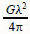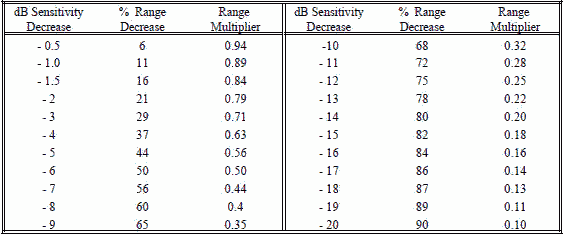;

Please Support RF Cafe by purchasing my  ridiculously low−priced products, all of which I created.

RF Workbench  (shareware)# Electronic Warfare and Radar Systems Engineering Handbook- One-Way Radar Equation / RF Propagation -

ONE-WAY RADAR EQUATION / RF PROPAGATION

The one-way (transmitter to receiver) radar equation is derived in this section. This equation is most commonly used in RWR or ESM type of applications. The following is a summary of the important equations explored in this section:Recall from Section 4-2 that the power density at a distant point from a radar with an antenna gain of Gt is the power density from an isotropic antenna multiplied by the radar antenna gain.

Power density from radar,If you could cover the entire spherical segment with your receiving antenna you would theoretically capture all of the transmitted energy. You can't do this because no antenna is large enough. (A two degree segment would be about a mile and three-quarters across at fifty miles from the transmitter.)

A receiving antenna captures a portion of this power determined by it's effective capture Area (Ae). The received power available at the antenna terminals is the power density times the effective capture area (Ae) of the receiving antenna.

For a given receiver antenna size the capture area is constant no matter how far it is from the transmitter, as illustrated in Figure 1. This concept is shown in the following equation:which is known as the one-way (beacon) equation        

In order to maximize energy transfer between an antenna and transmitter or receiver, the antenna size should  correlate λ/4. Control of beamwidth shape may become a problem when the size of the active element exceeds several wavelengths.The relation between an antenna's effective capture area (Ae) is:

Antenna Gain,or:  Equivalent Area, Ae =effective aperture is in units of length squared, s

proportional to wavelength. This physically means that to maintain the gain when doubling the frequency, the area is reduced by 1/4. This concept is illustrated in Figure 2.

If equation  is substituted into equation , the following relationship results:

Peak Power at Receiver Input = S (or PR) =is the signal calculated one-way from a transmitter to a receiver. For instance, a radar application might be to determine the signal received by a RWR, ESM, or an ELINT receiver. It is a general purpose equation and could be The free space travel of radio waves can, of course, be blocked, reflected, or distorted by objects in their path suchAs received signal power decreases by 1/4 (6 dB). This is due to the R2 term in equation . It illustrates a square on radius is decreased by 1/2, you further blow up the balloon, so the diameter or radius is doubled, the square has quadrupled in area.

The one-way free space loss factor (α1), (sometimes called the path loss factor) is given by the term 4πR2)(4π2) or (4πR /λ)2. As shown in Figure 3, the loss is due to the ratio of two factors (1) the effective radiated area of the transmit antenna, which is the surface area of a sphere (4πR2) at that distance (R), and (2) the effective capturearea (Ae) of the receive antenna which has a gain of one. If a receiving antenna could capture the whole surface area of the sphere, there would be no spreading loss, but a practical antenna will capture only a small part of the spherical radiation. Space loss is calculated using isotropic antennas for both transmit and receive, so α1 is independent of the actual antenna. Using Gr = 1 in equation  in section 3-1, Ae = λ/. Since this term is in the denominator of α1, the higher the frequency (lower λ) the more the space loss. Since Gt and Gr are part of the one-way radar equation, S (or Pr) is adjusted according to actual antennas as shown in the last portion of Figure 3. The value of the received signal (S) is:To convert this equation to dB form, it is rewritten as:( keep λ and R in same units)          

Since λ = c / f, equation  can be rewritten as:

10 Log (S or Pr) = 10 Log(PtGtGr) - α1               

Where the one-way free space loss, α1, is defined as:*        

The signal received equation in dB form is: 10log (Pr or S) = 10 log Pt + 10 log Gt + 10 log Gr - α1   

The one-way free space loss, α1, can be given in terms of a variable and constant term as follows:The value of f1 can be either in MHz or GHz as shown with commonly used units of R in the adjoining table.Note: To avoid having to include additional terms for these calculations, always combine any transmission line loss with antenna gain.

A value for the one-way free space loss (α1) can be obtained from:

(a) The One-way Free Space Loss graph (Figure 4). Added accuracy can be obtained using the

Frequency Extrapolation graph (Figure 5)

(b) The space loss nomograph (Figure 6 or 7)

(c) The formula for α1, equation .

FOR EXAMPLE:

Find the value of the one-way free space loss, α1, for an RF of 7.5 GHz at 100 NM.

(a) From Figure 4, find 100 NM on the X-axis and estimate where 7.5 GHz is located between the 1

and 10 GHz lines (note dot). Read α1 as 155 dB. An alternate way would be to read the α1 at

1 GHz (138 dB) and add the frequency extrapolation value (17.5 dB for 7.5:1, dot on Figure 5) to

obtain the same 155 dB value.

(b) From the nomogram (Figure 6), the value of α1 can be read as 155 dB (Note the dashed line).

(c) From the equation 11, the precise value of α1 is 155.3 dB.

Remember, α1 is a free space value. If there is atmospheric attenuation because of absorption of RF due to certain molecules in the atmosphere or weather conditions etc., the atmospheric attenuation is in addition to the space loss (refer to Section 5-1).Figure 4.  One-Way Free Space LossFigure 5. Frequency ExtrapolationFigure 6. One-Way Space Loss Nomograph For Distances Greater Than 10 Nautical MilesFigure 7. One-Way Space Loss Nomograph For Distances Less Than 10 Nautical MilesFigure 8. Visualization of One-Way Radar Equation

RWR/ESM RANGE EQUATION (One-Way)

The one-way radar (signal strength) equation  is rearranged to calculate the maximum range Rmax of RWR/ESM receivers. It occurs when the received radar signal just equals Smin as follows:In log form:

20 log Rmax = 10 log Pt + 10 log Gt - 10 log Smin - 20 log f + 20 log(c/4π)          

and since K1 = 20 log{4π/c times conversion units if not in m/sec, m, and Hz} (Refer to section 4-3 for values of K1).

10 log Rmax = ½[10 log Pt + 10 log Gt - 10 log Smin - 20 log f - K1] ( keep Pt and Smin in same units) 

If you want to convert back from dB, then Rmax � , where M dB is the resulting number in the brackets of equation 14.

From Section 5-2, Receiver Sensitivity / Noise, Smin is related to the noise factor S:

Smin = (S/N)min (NF)KToB            

The one-way RWR/ESM range equation becomes:RWR/ESM RANGE INCREASE AS A RESULT OF A SENSITIVITY INCREASE

As shown in equation  Smin-1 proportional to Rmax.

Therefore, -10 log Smin proportional to 20 log Rmax and the table below results:

% Range Increase: Range + (% Range Increase) x Range = New Range

i.e., for a 6 dB sensitivity increase, 500 miles +100% x 500 miles = 1,000 miles

Range Multiplier: Range x Range Multiplier = New Range i.e., for a 6 dB sensitivity increase

500 miles x 2 = 1,000 milesRWR/ESM RANGE DECREASE AS A RESULT OF A SENSITIVITY DECREASE

As shown in equation  Smin proportional to Rmax.

Therefore, -10 log Smin proportional to 20 log Rmax and the table below results:

% Range Decrease: Range - (% Range decrease) x Range = New Range

i.e., for a 6 dB sensitivity decrease, 500 miles - 50% x 500 miles = 250 miles

Range Multiplier: Range x Range Multiplier = New Range i.e., for a 6 dB sensitivity decrease

500 miles x .5 = 250 milesExample of One-Way Signal Strength: A 5 (or 7) GHz radar has a 70 dBm signal fed through a 5 dB loss

transmission line to an antenna that has 45 dB gain. An aircraft that is flying 31 km from the radar has an aft EW antenna with -1 dB gain and a 5 dB line loss to the EW receiver (assume all antenna polarizations are the same).

Note: The respective transmission line losses will be combined with antenna gains, i.e.:

-5 +45 = 40 dB, -5 - 1 = -6 dB, -10 + 5 = -5 dB.

(1) What is the power level at the input of the EW receiver?

Answer (1): Pr at the input to the EW receiver = Transmitter power - xmt cable loss + xmt antenna gain - space loss + rcvr antenna gain - rcvr cable loss.

Space loss (from section 4-3) @ 5 GHz = 20 log f R + K1 = 20 log (5x31) + 92.44 = 136.25 dB.

Therefore, Pr = 70 + 40 - 136.25 - 6 = -32.25 dBm @ 5 GHz (Pr = -35.17 dBm @ 7 GHz since α1 = 139.17 dB)

(2) If the received signal is fed to a jammer with a gain of 60 dB, feeding a 10 dB loss transmission line which is connected to an antenna with 5 dB gain, what is the power level from the jammer at the input to the receiver of the 5 (or 7) GHz radar?

Answer (2): Pr at the input to the radar receiver = Power at the input to the EW receiver+ Jammer gain - jammer cable loss + jammer antenna gain - space loss + radar rcvr antenna gain - radar rcvr cable loss .

Therefore, Pr = -32.25 + 60 - 5 - 136.25 + 40 = -73.5 dBm @ 5 GHz. (Pr = -79.34 dBm @ 7 GHz since

α1 = 139.17 dB and Pt = -35.17 dBm).

This problem continues in section 4-4, 4-7, and 4-10.

This HTML version may be printed but not reproduced on websites.

Related Pages on RF Cafe

- Introduction to Radar (Air University)

- Radar Techniques - Primer (1945 QST)